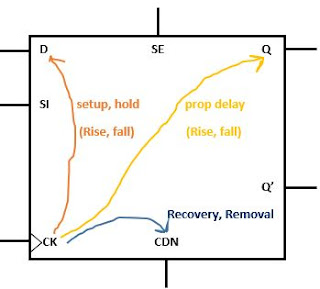## Friday, May 8, 2020

How the setup and hold checks are defined in the library?
Can both setup and hold be negative?

Sequential cells timing arcs:
Sequential cells timing arcs as shown in the figure.For synchronous inputs such as D, SI, SE there are following timing arcs
• Setup check arc (rising and falling)
• Hold check arc (rising and falling)

For asynchronous inputs such as CDN there are following timing arcs
• Recovery check arc
• Removal check arc

Synchronous checks: setup and hold
The setup and hold timing checks are needed to check the proper propagation of data through the sequential circuits. These timing checks are used to verify the data input (D) is unambiguous at the active edge of the clock so the proper data is latched at the active edge. These timing checks verify the data input is stable before and after the clock.

The setup and hold timing constraints for the synchronous pin of a sequential cell described in terms of two-dimensional table as shown in the figure.
The setup and hold timing constraints on the input pin D with respect to the rising (active) edge of clock CK of the FF.

The two-dimensional models are in terms of data and clock transition time at the constrained_pin (Data pin D) and the related_pin (clock pin CK) respectively. Index 1 is showing data transition (D) at the rising edge and index 2 is showing the clock transition (CK) at the rising edge. Thus with data D pin rise transition of 0.4ns and clock CK pin rise transition of 0.84ns so the setup constraint for the rising edge of the D pin is 0.112ns  (this value is read from the rise_constraint table).

For the falling edge of the D pin, the setup constraint will tool from the fall_constraint table of setup.
Rise_constraint and fall_constraint tables of the setup constraint refer to the constrained_pin. The clock transition used is determined by the timing_type which specifies whether the cell is rising edge-triggered or falling edge-triggered.

Negative values in setup and hold checks:
In the last post I mentioned the reason for negative values in the library. We can consider a flip flop like a black box and we don't know what is inside the flop. Just we can assume sometimes data reach early at the data pin than the clock reaches at clock pin or sometimes clock reaches early at the clock pin than the data at data pin.

We notice that some of the hold values in the fig are negative. This normally happens when the path from the pin of the Flop to the internal latch point for the data is longer than the corresponding path for the clock. Thus a negative hold timing check implies that the data pin (D) of the flop can change before the clock pin (CK) and still meet hold time check.

The setup values of a FF can also be negative and this means that at the pins of the flip flop, the data can change after the clock pin and still, meet the setup check.

Can both setup and hold be negative? This ques is related to the library file.
No, For the setup and hold checks to be consistent and proper, the sum of setup and hold values should be positive. If there is one of the setup (or hold) check contains negative values –then corresponding hold (or setup) should be sufficiently positive so that the setup plus hold value is a positive quantity. so the data will proper in the setup and hold window.
The setup plus hold time is the width of the region where the data signal is required to be stable.
For flip flops, it is helpful to have a negative hold time on scan data input pins. This gives the flexibility in terms of clock skew and can eliminate the need for almost all the  buffer insertion for fixing hold violations in scan mode (scan mode is the one in which flip flop are tied serially forming a scan chain – output of flip flop is typically connected to the scan data input pin of the next flip flop in series, these connections are used for testability)